# Insert Math in Word with with Equation Editor

Microsoft Word includes an Equation Editor which can be used to insert common mathematical expressions in the document. These equations are converted to accessible Math when the document is converted to EPUB format using WordToEPUB.

## Insert new equations

To type a new equation, press Alt + = on your keyboard. Alternatively, On the Insert tab, click on Symbols and then click on Equation.

An equation placeholder will be inserted in the document inside which you can type the equation. You can also choose from numerous equations available in the gallery which can be seen in the Equation dropdown.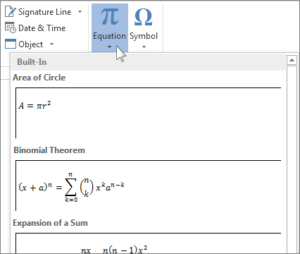After you insert the equation the Equation Tools Design tab opens with symbols and structures that can be added to your equation.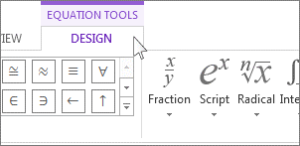## Editing existing equations

1. Select the equation, the Equation Tools will become available in the ribbon.
2. Choose Design to see tools for adding various elements to your equation. You can add or change the following elements.
• Math related symbols are available in the Symbols group. To see all the symbols, click the More button. To see other sets of symbols, click the arrow in the upper right corner of the gallery.
• The Structures group provides structures you can insert. Just choose a structure to insert it and then replace the placeholders, the small dotted-line boxes, with your own values.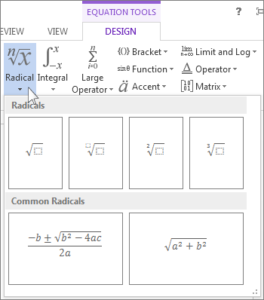• The Professional option displays the equation in a professional format optimized for display. The Linear option displays the equation as source text, which can be used to make changes to the equation if needed. The linear option will display the equation in either UnicodeMath format, or LaTeX format, which can be set in the Conversions chunk.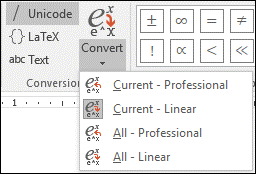• It is possible to convert all equations in a document to the Professional or Linear formats, or a single equation only, if the math zone is selected or the cursor is in the equation.

## Equations on touch- and pen-enabled devices

You can write equations using a stylus or your finger on such devices.

1. In the Equation Tab, click on Ink Equation
2. Use a stylus or your finger to write a math equation by hand. If you’re not using a touch device, use your mouse to write out the equation. You can select portions of the equation and edit them as you go, and use the preview box to make sure Word is correctly interpreting your handwriting.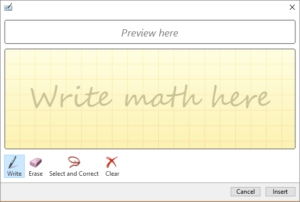3. When you’re satisfied, click Insert to convert the ink equation to an equation in your document.